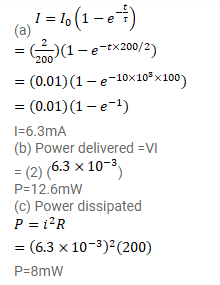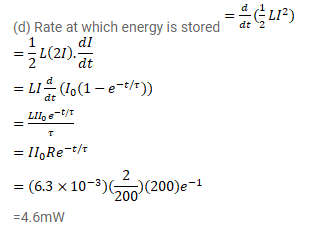# An inductor of inductance 2.00H is joined in series

Question:

An inductor of inductance $2.00 \mathrm{H}$ is joined in series with a resistor of resistance $200 \Omega$ and a battery of emf $2.00 \mathrm{~V}$. At $t=10 \mathrm{~ms}$, find

(a) the current in the circuit,

(b) the power delivered by the battery,

(c) the power dissipated in heating the resistor and

(d) the rate at which energy is being stored in magnetic field.

Solution: